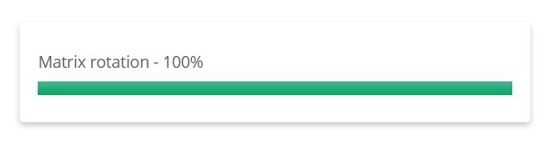## Problem Statement :

Given a non empty array inarr consisting of integers seperated by ‘,'(comma), print the output based on the below logic:

Assume that inarr will have atleast 4 elements also last element of inarr will always be a positive non zero integer

## Question :## Code Solution :

```				```
import math

M = 0
matrix = []

def rotateMatrix(k):
global M, matrix
temp =  * M
k = k % M
for i in range(0, M):

for t in range(0, M - k):
temp[t] = matrix[i][t]

for j in range(M - k, M):
matrix[i][j - M + k] = matrix[i][j]

for j in range(k, M):
matrix[i][j] = temp[j - k]

def solve(s):
global M, matrix
l = s.split(",")
for i in range(len(l)):
l[i] = int(l[i])

m = int(math.sqrt((len(l))))
size = m * m
start = 0
high = -1e6
for i in range(len(l) - size, -1, -1):
right = 0
left = 0
for j in range(0, m):
p = i + (m + 1) * j
right = right + l[p]
for j in range(1, m + 1):
p = i + (m - 1) * j
left = left + l[p]
if right >= high:
start = i
high = right
if left >= high:
start = i
high = left
ans = []
for i in range(0, m):
q = []
for j in range(0, m):
q.append(l[start])
start = start + 1
ans.append(q)
M = m
matrix = ans
rotateMatrix(l[len(l) - 1])
ans = []

for i in range(0, m):
for j in range(0, m):
if j==m-1:
print(matrix[i][j])
else:
print(matrix[i][j],end=" ")

s = input();
solve(s)
```
```